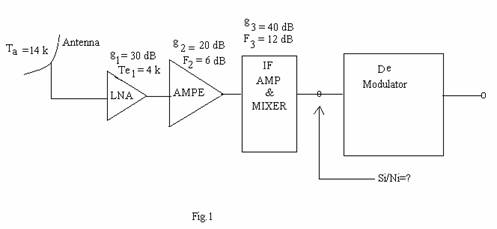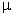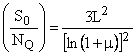Time: 3 Hours                                                                                                     Max. Marks: 100

NOTE: There are 9 Questions in all.

·      Question 1 is compulsory and carries 20 marks. Answer to Q. 1. must be written in the space provided for it in the answer book supplied and nowhere else.

·      Out of the remaining EIGHT Questions answer any FIVE Questions. Each question carries 16 marks.

·      Any required data not explicitly given, may be suitably assumed and stated.

Q.1       Choose the correct or best alternative in the following:                                         (2x10)

a.       In a TV receiver, the circuit that separates sync pulses from the composite video signal is

(A)  a differentiator                              (B)   an integrator

(C)    the keyed ACC amplifier             (D)  a clipper

b.      The quantization noise in a PCM system depends upon

(A)    Sampling rate only

(B)    number of quantization levels only

(C) both the sampling rate and number of quantization levels only.

(D) none of the above is correct.

c.       In FM, the frequency deviation is

(A)    Constant.

(B)    proportional to modulating frequency.

(C)    zero.

(D)    Proportional to amplitude of modulating signal.

d.   To compare the noise performance of the receiver, which of the following quantities is not used?

(A)    Noise figure.                               (B) Noise temperature.

(C)  equivalent noise resistance.           (D) input noise voltage.

e.   The carrier and modulating frequency are 100 kHz and 5 kHz respectively. The bandwidth of the AM signal will be

(A)     10 kHz.                                       (B)  2 kHz.

(C)  5 kHz.                                          (D)  40 kHz.

f.    A balanced modulator is used mainly

(A)     to limit noise picked up by receiver.

(B)     To suppress carrier signal in order to create a single sideband or double sideband.

(C)     To produce balanced modulation of carrier wave

(D)    To produce 100 percent modulation.

g.   A solution to the ‘blind speed’ problem in MTI radar is to

(A)     Change the Doppler frequency.    (B)  vary the PRF

(C)  use monopulse                              (D)  use MTI

h.   A forward error-correcting code corrects errors by

(A)    Requiring partial retransmission of the signal.

(B)    Requiring retransmission of the signal.

(C)    Requiring no part of the signal to be transmitted.

(D)    Using parity to correct the errors in all cases.

i.    If the peak transmitted power in a radar system is increased by a factor of 16,  the maximum range will be increased by a factor of

(A)   2                                                  (B) 4

(C) 8                                                   (D) 16

j.    Phase ambiguity in BPSK demodulators, can cause an error rate of

(A)  50%                                             (B)  75%

Answer any FIVE Questions out of EIGHT Questions.

Each question carries 16 marks.

Q.2     a.   Describe the method for calculation of noise figure of a receiver with the help of a block diagram and discuss the procedural steps for the same.        (6)

b.   For the receiver shown in Fig.1.find

(i)                  equivalent noise temperature of the receiver.

(ii)                noise figure of the receiver and

(iii)               If the bandwidth of signal is 36 MHz and available signal power at antenna input is 0.1 pw, find Si/Ni (SNR) at input  to the demodulator.                                            (6)c.   Justify the need of modulated carrier for the transmission of messages in the radio channel.              (4)

Q.3     a.   Explain a method of generating a single sideband signal using balanced modulators and phase shifters with neat sketch.                                          (6)

b.   A baseband signal m(t) is transmitted by using DSB-SC. The power spectral density of m(t) isFind (i) input signal power. (ii) Output signal power and (iii) output signal to noise ratio.                   (6)

c.   Derive the formula for the figure of merit for an AM-Demodulator.                      (4)

Q.4     a.   Describe the role of balanced FM demodulator for reducing the distortion produced by the non linearity of the bandpass filters with neat sketch and necessary equations.                                            (6)

b.   Discuss the need of preemphasis and deemphasis in commercial FM broadcasting and justify that the use of the same significantly improves the output signal to noise ratio.                                            (6)

c.   Compare AM and FM under the constraint of similar signal power, noise power spectral density and baseband bandwidth.                           (4)

Q.5       a.   When a-law compander is used in PCM, the output signal to quantizing noise ratio for>>1 is approximated by. Derive the 6 dB rule for=255.                         (6)

b.   The T1 carrier system used in digital telephony multiplexes 24 voice channels based on 8-bit PCM. Each voice signal is usually put through a low-pass fitter with the cut off frequency of about 3.4 kHz. The filtered voice signal is sampled at 8 kHz. In addition a single bit is added at the end of the frame for the purpose of synchronization. Calculate (i) the duration of each bit (ii) the resultant transmission rate and (iii) the minimum required transmission bandwidth.                                                                        (6)

c.   Briefly describe the QPSK demodulator.                                                            (4)

Q.6     a.   Draw the signal space diagram for QPSK and derive the error probability for QPSK.                     (6)

b.   Compare DM and ADM in brief.                                                                       (6)

c.   A DM system is designed to operate at 3 times the Nyquist rate for a signal with a 3-kHz bandwidth. The quantizing step size is 250 mV.

(i)                  Determine the maximum amplitude of a 1-kHz input sinusoid for which the delta modulator does not show slope overload.

(ii)                Determine the postfiltered output signal to quantizing noise ratio for the signal to part (i).                                   (4)

Q.7     a.   “For finite signal and noise power channel capacity always remains finite”. Justify.               (6)

b.   Derive the RADAR range equation and discuss briefly the performance parameters for the RADAR.                                                                     (6)

c.   A high resolution black and white TV picture consists of about 2 x 106  picture elements and 16 different brightness levels. Pictures are repeated at the rate of 32 per second. All picture elements are assumed to be independent, and all levels have equal likelihood of occurrence. Calculate the average rate of information conveyed by this TV picture source.     (4)

Q.8     a.   Consider a generator matrix G for a non-systematic (6,3) codeConstruct the code for this G when the data word is 001? Show that dmin, the minimum distance between code words is 3. What is its error correcting capability?                                                     (6)

b.   What do you understand by phase error in color tranmission? Explain fully

how it is cancelled in the PAL color system. Why are color burst pulses

sent alongwith other sync pulses?                                                                        (6)

c.  Mention the limitations of an MTI RADAR.                                                         (4)

Q.9           Write notes on any TWO of the following:

(i)                  Channel capacity of a Gaussian channel.